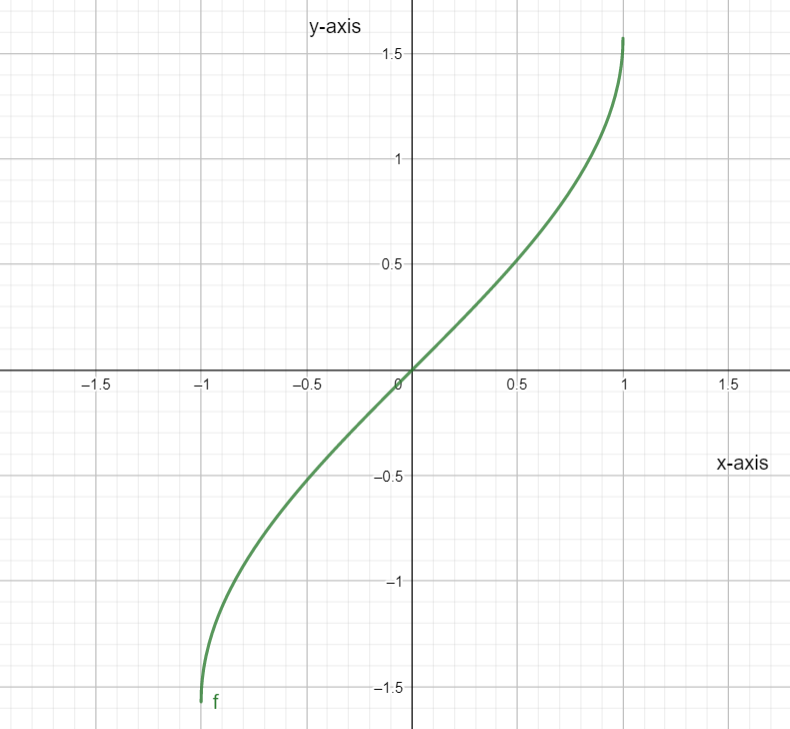Courses
Courses for Kids
Free study material
Offline Centres
MoreLast updated date: 04th Dec 2023
Total views: 280.8k
Views today: 8.80k

# Write a range of $f\left( x \right)={{\sin }^{-1}}x$ other than $\left[ -\dfrac{\pi }{2},\dfrac{\pi }{2} \right]$Verified
280.8k+ views
Hint: To find the range of given function other than the given range we will plot its graph and check its value in other points. Firstly we will draw a graph of a given function then we will see under what range its value falls. Then we will take a range accordingly and get our desired answer.

The function given to us is as follows:
$f\left( x \right)={{\sin }^{-1}}x$
The range is provided to us as follows:
$\left[ -\dfrac{\pi }{2},\dfrac{\pi }{2} \right]$
We will find value of the function in this range as follows:
So at $x=-\dfrac{\pi }{2}$ we get the value as:
\begin{align} & f\left( \dfrac{\pi }{2} \right)={{\sin }^{-1}}\left( -\dfrac{\pi }{2} \right) \\ & \Rightarrow f\left( \dfrac{\pi }{2} \right)=-{{\sin }^{-1}}\left( \dfrac{\pi }{2} \right) \\ & \Rightarrow f\left( \dfrac{\pi }{2} \right)=-1 \\ \end{align}
So at $x=\dfrac{\pi }{2}$ we get the value as:
\begin{align} & f\left( \dfrac{\pi }{2} \right)={{\sin }^{-1}}\left( \dfrac{\pi }{2} \right) \\ & \Rightarrow f\left( \dfrac{\pi }{2} \right)=1 \\ \end{align}
So the value of function $f\left( x \right)={{\sin }^{-1}}x$ lies in $\left[ -1,1 \right]$
We get the graph of the function as below:So we have to take a range that gives the value under the above graph.
So we can take the range as $\left[ \dfrac{\pi }{2},\dfrac{3\pi }{2} \right]$
Hence range of $f\left( x \right)={{\sin }^{-1}}x$ other than $\left[ -\dfrac{\pi }{2},\dfrac{\pi }{2} \right]$ is $\left[ \dfrac{\pi }{2},\dfrac{3\pi }{2} \right]$

Note: Trigonometric is a branch of mathematics that studies the relation between the side lengths and the angles of a triangle. There are six types of trigonometric functions which are sine, cosine, tangent, secant, cosecant and cotangent. As the six trigonometric functions are periodic in nature they are not injective and hence they are invertible by restricting the domain of the function. The graph of the inverse of the sine function is like a reflection over the line $y=x$ of the sine function. Sometimes we write the inverse function as $\arcsin \left( x \right)$ because the superscript $-1$ is not an exponent so to avoid any confusion a different notation can be used.